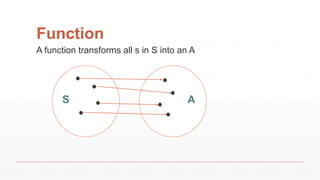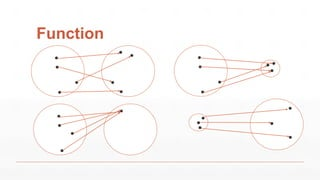Ce diaporama a bien été signalé.
Le téléchargement de votre SlideShare est en cours. ×

# Beyond Scala Lens

Publicité
Publicité
Publicité
Publicité
Publicité
Publicité
Publicité
Publicité
Publicité
Publicité
PublicitéChargement dans…3
×

## Consultez-les par la suite

1 sur 67 Publicité

# Beyond Scala Lens

A Lens is a functional concept which solves a very common problem: how to update a complex immutable structure. This is probably the reason why Lenses are relatively well known in functional programming languages such as Haskell or Scala. However, there are far less resources available on the generalization of Lenses known as "optics".

In this slides, I would like to go through a few of these optics namely Iso, Prism and Optional, by showing how they relate to each other as well as how to use optics in a day to day programming job.

A Lens is a functional concept which solves a very common problem: how to update a complex immutable structure. This is probably the reason why Lenses are relatively well known in functional programming languages such as Haskell or Scala. However, there are far less resources available on the generalization of Lenses known as "optics".

In this slides, I would like to go through a few of these optics namely Iso, Prism and Optional, by showing how they relate to each other as well as how to use optics in a day to day programming job.

Publicité
Publicité

### Beyond Scala Lens

1. 1. Beyond Scala Lenses github: @julien-truffaut or twitter: @JulienTruffaut
2. 2. Function S A A function transforms all s in S into an A
3. 3. Function
4. 4. Isomorphism S A For all s: S, g(f(s)) == s For all a: A, f(g(a)) == a f g
5. 5. Iso case class Iso[S,A]( get : S => A, reverseGet: A => S ) Properties: For all s: S, reverseGet(get(s)) == s For all a: A, get(reverseGet(a)) == a
6. 6. Modify A A f
7. 7. Modify A A S S f get reverseGet modify
8. 8. Compose S A Iso B Iso Iso
9. 9. Iso Derived Methods case class Iso[S,A]( get : S => A, reverseGet: A => S ){ def modify(m: A => A): S => S def reverse: Iso[A,S] def compose[B](other: Iso[A,B]): Iso[S,B] }
10. 10. Units class Robot{ def moveBy(d: Double): Robot } val nono: Robot = … nono.moveBy(100.5) // Meters nono.moveBy(3) // Kilometers nono.moveBy(-2.5) // Yards
11. 11. Safe Unit case class Meter(d: Double) case class Yard(d: Double) class Robot{ def moveBy(m: Meter): Robot } nono.moveBy(Meter(100.5)) nono.moveBy(10) // does not compile nono.moveBy(Yard(3.0)) // does not compile
12. 12. Iso Meter Yard
13. 13. Meter to Yard val meterToYard: Iso[Meter, Yard] = Iso( m => Yard(m.value * 1.09361), y => Meter(y.value / 1.09361) ) meterToYard.get(Meter(200)) == Yard(218.7219999…) nono.moveBy(meterToYard.reverseGet(Yard(2.5))
14. 14. Iso Meter Yard Kilometer
15. 15. Iso Meter Yard Kilometer Mile
16. 16. Iso Composition case class Kilometer(value: Double) case class Mile(value: Double) val meterToKilometer: Iso[Meter, Kilometer] = … val yardToMile : Iso[Yard, Mile] = … val kilometerToMile: Iso[Kilometer, Mile] = meterToKilometer.reverse compose meterToYard compose yardToMile
17. 17. Different Representation val stringToList: Iso[String, List[Char]] = Iso(_.toList, _.mkString(“”)) def listToVector[A]: Iso[List[A], Vector[A]] = Iso(_.toVector, _.toList)
18. 18. Generic Programming case object Red val red: Iso[Red, Unit] = Iso(Red => (), () => Red) case class Person(name: String, age: Int) val personToTuple: Iso[Person, (String, Int)] = …
19. 19. Iso Properties For all s: S, reverseGet(get(s)) == s For all a: A, get(reverseGet(a)) == a
20. 20. Scalacheck def isoLaws[S,A](iso: Iso[S,A]) = new Properties { property(“One Way”) = forAll { s: S => iso.reverseGet(iso.get(s)) == s } property(“Other Way”) = forAll { a: A => iso.get(iso.reverseGet(a)) == a } }
21. 21. Scalacheck import org.spec2.scalaz.Spec class IsoSpec extends Spec { checkAll(“meter to yard”, isoLaws(meterToYard)) } val meterToYard: Iso[Meter, Yard] = Iso( m => Yard(m.value * 1.09361), y => Meter(y.value / 1.09361) )
22. 22. Scalacheck A counter-example is: [Yard(1.518707721E9)] (after 24 tries) scala> meterToYard.get(meterToYard.reverseGet( Yard(1.518707721E9))) scala> res0: Yard= Yard(1.5187077210000002E9)
23. 23. Relaxed Isomorphism S A For all s: S such as f(s) exists, g(f(s)) == s For all a: A, f(g(a)) == a ? f g f is a Function[S, Option[A]] g is a Function[A, S]
24. 24. Prism case class Prism[S,A]( getOption : S => Option[A], reverseGet: A => S ) Properties: For all s: S, getOption(s) map reverseGet == Some(s) || None For all a: A, getOption(reverseGet(a)) == Some(a)
25. 25. Pattern matching / Constructor sealed trait List[A] case class Cons[A](h: A, t: List[A]) extends List[A] case class Nil[A]() extends List[A] Cons.unapply(List(1,2,3)) == Some((1, List(2,3))) Cons.unapply(Nil) == None Cons.apply(1, List(2,3)) == List(1,2,3)
26. 26. Prism sealed trait List[A] case class Cons[A](h: A, t: List[A]) extends List[A] case class Nil[A]() extends List[A] def cons[A]: Prism[List[A], (A, List[A])] = … cons.getOption(List(1,2,3)) == Some((1, List(2,3))) cons.getOption(Nil) == None cons.reverseGet(1, List(2,3)) == List(1,2,3)
27. 27. Prism Derived Methods case class Prism[S,A]( getOption: S => Option[A], reverseGet: A => S ){ def isMatching(s: S): Boolean def modify(f: A => A): S=> S def modifyOption(f: A => A): S => Option[S] def compose[B](other: Prism[A,B]): Prism[S,B] def compose[B](other: Iso[A,B]): ???[S,B] }
28. 28. Iso – Prism Optic f g Iso S => A A => S Prism S => Option[A] A => S def isoToPrism[S,A](iso: Iso[S,A]): Prism[S,A] = Prism( getOption = s => Some(iso.get(s)), reverseGet = iso.reverseGet )
29. 29. Iso – Prism case class Prism[S,A]{ def compose[B](other: Prism[A,B]): Prism[S,B] def compose[B](other: Iso[A,B]): Prism[S,B] } case class Iso[S,A]{ def compose[B](other: Iso[A,B]): Iso[S,B] def compose[B](other: Prism[A,B]): Prism[S,B] }
30. 30. Enum sealed trait Day case object Monday extends Day case object Tuesday extends Day val tuesday: Prism[Day, Unit] = … tuesday.getOption(Monday) == None tuesday.getOption(Tuesday) == Some(()) tuesday.reverseGet(()) == Tuesday
31. 31. Json sealed trait Json case class JNumber(v: Double) extends Json case class JString(s: String) extends Json val jNum: Prism[Json, Double] = … jNum.modify(_ + 1)(JNumber(2.0)) == JNumber(3.0) jNum.modify(_ + 1)(JString(“”)) == JString(“”) jNum.modifyOption(_ + 1)(JString(“”)) == None
32. 32. Safe Down Casting val doubleToInt: Prism[Double, Int] = … doubleToInt.getOption(3.4) == None doubleToInt.getOption(3.0) == Some(3) doubleToInt.reverseGet(5) == 5.0
33. 33. Prism Composition sealed trait Json case class JNumber(v: Double) extends Json case class JString(s: String) extends Json val jInt: Prism[Json, Int] = jNum compose doubleToInt jInt.getOption(JNumber(3.0)) == Some(3) jInt.getOption(JNumber(5.9)) == None jInt.getOption(JString(“”)) == None
34. 34. Where is the bug? def stringToInt: Prism[String, Int] = Prism( getOption = s => Try(s.toInt).toOption, reverseGet = _.toString ) stringToInt.modify(_ * 2)(“5”) == “10” stringToInt.getOption(“5”) == Some(5) stringToInt.getOption(“-3”) == Some(-3) stringToInt.getOption(“5.7”) == None stringToInt.getOption(“99999999999999999”) == None stringToInt.getOption(“Hello”) == None
35. 35. Tadam ! ” .toInt = 9“
36. 36. Prism S A B COr Or sealed trait S class A extends S class B extends S class C extends S
37. 37. Lens S A B CAnd And case class S(a: A, b: B, c: C)
38. 38. Lens case class Lens[S,A]( get: S => A, set:(A, S) => S ) Properties: For all s: S, set(get(s), s) == s For all a: A, s: S, get(set(a, s)) == a
39. 39. Iso – Lens Optic f g Iso S => A A => S Lens S => A (A, S) => S def isoToLens[S,A](iso: Iso[S,A]): Lens[S,A] = Lens( get = iso.get, set = (a, _) => iso.reverseGet(a) )
40. 40. Accessors case class Person(name: String, age: Int) val age = Lens[Person, Int](_.age, (a, p) => p.copy(age = a)) val zoe = Person(“Zoe”, 25) age.get(zoe) == 25 age.set(20, zoe) == Person(“Zoe”, 20) age.modify(_ + 1)(zoe) == Person(“Zoe”, 26)
42. 42. Tuples def first[A,B]: Lens[(A,B), A] = … def second[A,B]: Lens[(A,B), B] = … val tuple = (2,“Zoe”) first.set(4, tuple) == (4,“Zoe”) second.modify(_.reverse)(tuple) == (2,“eoZ”)
43. 43. Universal Case Class Accessors case class Person(name: String, age: Int) val personToTuple: Iso[Person,(String,Int)] = … val zoe = Person(“Zoe”, 25) (personToTuple compose second).set(30, zoe) == Person(“Zoe”, 30)
44. 44. At def at[K,V](k: K): Lens[Map[K,V], Option[V]] = Lens( get = m => m.get(k), set = (optV, m) => optV match { case None => m – k case Some(v)=> m + (k -> v) } )
45. 45. Map 1“one” 2“two” 1“one” at(“three”).set(Some(3), m) 1“one” 2“two” at(“two”).set(None, m) 3“three”
46. 46. What Next? Optic f g Iso S => A A => S Prism S => Option[A] A => S Lens S => A (A, S) => S
47. 47. Optional Optic f g Iso S => A A => S Prism S => Option[A] A => S Lens S => A (A, S) => S Optional S => Option[A] (A, S) => S
48. 48. Optional PrismLens Iso
49. 49. Optional case class Optional[S,A]( getOption: S => Option[A], set :(A, S) => S ) Properties: For all s: S, getOption(s) map set(_, s) == Some(s) For all a: A, s: S, getOption(set(a, s)) == Some(a) || None
50. 50. Head def cons[A]: Prism[List[A], (A, List[A])] = … def first[A, B]: Lens[(A, B), A] = … def head[A]: Optional[List[A], A] = cons compose first head.getOption(List(1,2,3)) = Some(1) head.set(0, List(1,2,3)) = List(0,2,3)
51. 51. Void def void[S,A]: Optional[S, A] = Optional( getOption = s => None, set = (a, s) => s ) void.getOption(“Hello”) = None void.set(1,“Hello”) = “Hello” void.setOption(1,“Hello”) = None
52. 52. Index def index[A](i: Int): Optional[List[A], A] = if(i < 0) void else if(i == 0) head else cons compose second compose index(i – 1) index(-1).getOption(List(1,2,3)) == None index(1).getOption(List(1,2,3)) == Some(2) index(5).getOption(List(1,2,3)) == None index(1).set(10, List(1,2,3)) == List(1,10,3)
53. 53. Index for Vector def vectorToList[A]: Iso[Vector[A], List[A]] = … def indexV(i: Int): Optional[Vector[A], A] = vectorToList compose index(i)
54. 54. Index != At 3 5 6 2 0 1 2 3 3 5 99 2 0 1 2 3 index(2).set(99, l) val l = List(3,5,6,2) 3 5 6 2 ? ? ? 99 0 1 2 3 4 5 6 7 3 5 6 2 0 1 2 3 index(7).set(99, l)
55. 55. Study Case: Http Request sealed trait Method case object GET extends Method case object POST extends Method case class URI( host: String, port: Int, path: String, query: Map[String, String] ) case class Request( method: Method, uri: URI, headers: Map[String, String], body: String )
56. 56. Study Case: Http Request val r = Request( method = GET, uri = URI(“localhost”,8080,“/ping”,Map(“age”->“15”)), headers = Map.empty, body = “” )
57. 57. Which method? val method: Lens[Request, Method] = … val GET: Prism[Method, Unit] = … method.get(r) // GET (method compose GET).isMatching(r) // true
58. 58. How to update host? val uri: Lens[Request, URI] = … val host: Lens[URI, String] = … (uri compose host).set(“foo.io”)(r) Request( method = GET, uri = URI(“foo.io”,8080,“/ping”,Map(“age”->“15”)), headers = Map.empty, body = “” )
59. 59. How to increase age query? val query: Lens[URI, Map[String, String]] = … (uri compose query compose index(“age”) compose stringToInt ).modify(_ + 1)(r) Request( method = GET, uri = URI(“localhost”,8080,“/ping”,Map(“age”->“16”)), headers = Map.empty, body = “” )
60. 60. How to add header? val headers: Lens[Request, Map[String, String]] = … (headers compose at(“Content-Length”)).set(Some(“0”))(r) Request( method = GET, uri = URI(“localhost”,8080,“/ping”,Map(“age”->“15”)), headers = Map(“Content-Length”->“0”), body = “” )
61. 61. More power!!! val r2 = Request( method = POST, uri = URI(“localhost”,8080,“/pong”,Map.empty), headers = Map(“x-custom1”->“5”, “x-custom2”->“10”), body = “Hello World” )
62. 62. Traversal (headers compose filterIndex(_.startsWith(“x-”)) compose stringToInt ).modify(_ * 2)(r2) Request( method = POST, uri = URI(“localhost”,8080,“/pong”,Map.empty), headers = Map(“x-custom1”->“10”, “x-custom2”->“20”), body = “Hello World” )
63. 63. Optional PrismLens Iso Traversal Setter Getter Fold
64. 64. Monocle goodies ▪ Provides lots of built-in optics and functions ▪ Macros for creating Lenses, Iso and Prism ▪ Syntax to use optics as infix operator ▪ Experimental state support
65. 65. Resources ▪ Monocle on github ▪ Simon Peyton Jones’s lens talk at Scala Exchange 2013 ▪ Edward Kmett on Lenses with the State Monad
66. 66. Acknowledgement ▪ Member Monocle and Cats gitter channel for advice and review ▪ Special thanks to Ilan Godik (@NightRa) for helping with slides and content
67. 67. Thank you!